﻿ MotionDesigner Reference & User Interface > Motion Laws > CV Inverse Crank [Special]

# CV Inverse Crank

Navigation:  MotionDesigner Reference & User Interface > Motion Laws >

# CV Inverse Crank

### Constant-Velocity Inverse Crank

CV (Constant Velocity) Inverse Crank is similar to the Y–Inverse Sinusoid Motion-Law.

We urge you to use this motion-law in preference to the Y-Inverse Sinusoid.

This segment can be used more than once in one machine cycle, whereas the Y-Inverse-Sinusoid can be used only one time.

This segment is best explained by considering the X and Y motion components of a Point that is at the end of a rotating-Part or Crank.

When a Crank rotates with Constant angular velocity, the motion of the Point at the end of the Crank is Simple-Harmonic-Motion when it is projected onto a Line (a Sine function).

When you apply the CV-Inverse-Crank segment to a Crank, the motion of a Point at the end of the Crank that is projected onto a Line can be made to move with a constant linear velocity for a phase of the Crank's rotation.

SEGMENT-EDITOR

See the image Segment-Parameters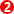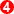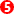below

You can set the:

Minimum Velocity: or the Angle, relative to the Base-Value of the Minimum-Velocity

Rel Start: the angle relative toRel End: the angle relative toIf you use the Flexible-Polynomial Segment before and after this segment, you can:

Use the Blend Control Button to make the End of the Flexible-Polynomial Segment match the start of this Segment.

Use the Blend Control Button to make the Start of the Flexible-Polynomial Segment match the end of this Segment.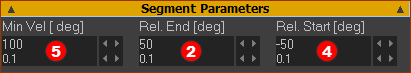Segment-Parameters of the 'Constant-Velocity Inverse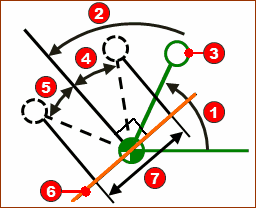#### Segment Editor : Segment-Parameters

Minimum Velocity: the Angle of Minimum Velocity

This is the angle, after the Base-Value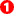* of the Crank, at which the angular velocity of the Crank is a minimum. At all other angles, within the range of this segment, the angular velocity of the Crank is greater than the Minimum Velocity.

The direction of the Constant-Velocity vector for Point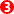on the Crank is projected onto an imaginary Line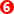, which is perpendicular to the Angle of Minimum Velocity.

*The Base-Valueis the parameter defined in the Motion-Dimension FB dialog for the Crank, not by the position of the End of the Previous-Segment.

Rel. Start: the Relative Angle before the Minimum Velocity Angle

The angle, before from the Angle of Minimum Velocity, at which the Constant-Velocity of Pointstarts.

Rel. End: the Relative Angle after the Minimum Velocity Angle

The angle, after the Angle of Minimum Velocity, at which the Constant-Velocity of Pointends.

Note:

The Crank rotates Counter-Clockwise when Rel. Start is a Negative #  and Rel. End is a Positive #

The Crank rotates Clockwise when Rel. Start is a Positive #  and Rel. End is a Negative #

The velocity of the Pointwhen projected on to the Lineis constant while the crank moves within the angular range of:

Start Angle =+-...to...

End Angle =++The linear velocity and the length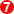of the Constant-Velocity of the Point along the line is a function of the:

Segment-Width

Length of the Rocker/Crank

Segment-Parameters: Rel Start Angle and Rel End Angle.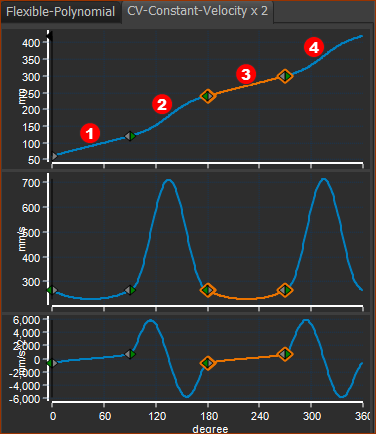CV Inverse-Crank2 x

An example motion with four segments and two CV Inverse Crank

Segment 1: Constant-Velocity Inverse-Crank

X-axis : 0 to 90º

Segment Parameters are:

Angle of Minimum Velocity = 90º

Relative Start Angle = -30º

Relative End = 30º

With these Parameters:

When X-axis = 0º ; Y–axis = 60º.

When X-axis =90 ; Y–axis = 120º

Segment 2: Flexible-Polynomial

X-axis : 90 to 180º

Y-axis at Start P=120. V, A, J flow from the end of the Constant-Crank-Velocity Segment.

Y-axis at End 240º

Segment 3: Constant-Velocity Inverse-Crank

X-axis : 180 to 270º

Segment Parameters are:

Angle of Minimum Velocity = 270º

Relative Start Angle = -30º

Relative End = 30º

With these Parameters:

When X-axis = 180º ; Y–axis = 240º

When X-axis =270 ; Y–axis = 300º

Segment 4: Flexible-Polynomial

X-axis : 90 to 180º

Y-axis P at Start = 300º. V, A, J flow from the end of the Constant-Crank-Velocity Segment.

Y=axis at End = 420º.